#### You may also like### Doodles

Draw a 'doodle' - a closed intersecting curve drawn without taking pencil from paper. What can you prove about the intersections?### Russian Cubes

I want some cubes painted with three blue faces and three red faces. How many different cubes can be painted like that?### Areas and Ratios

Do you have enough information to work out the area of the shaded quadrilateral?

# Calculating with Cosines

#### If you are a teacher, click here for a version of the problem suitable for classroom use, together with supporting materials. Otherwise, read on...

If I tell you two sides of a right-angled triangle, you can easily work out the third using Pythagoras' theorem. But what if the angle between the two sides is not a right angle? Is there a way to work out the length of the third side?

Some students tried to work out a formula to work out the length of the third side when we know two sides and the angle between them.

Below, you can see the start of their methods. Can you finish each method to produce a formula?

Student 1: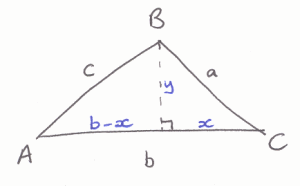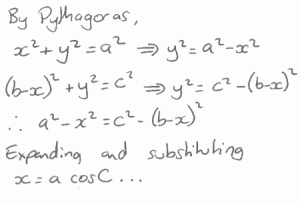Student 2: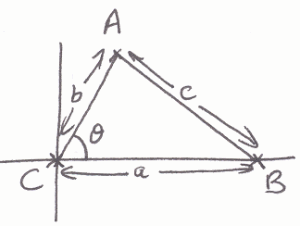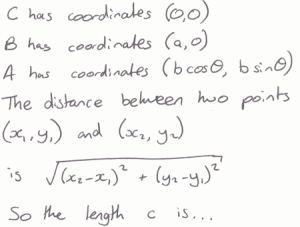Student 3: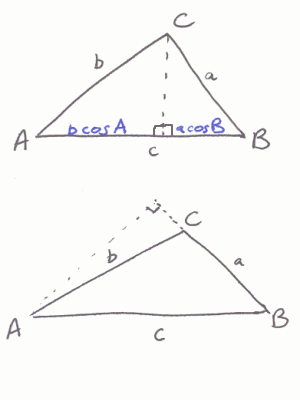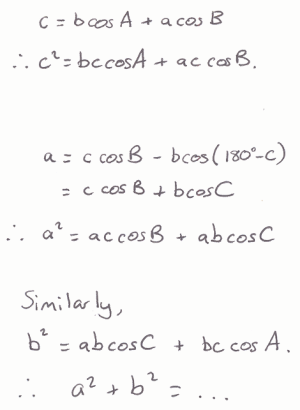Does each method lead to an equivalent formula?

Does each method work for both acute-angled and obtuse-angled triangles?